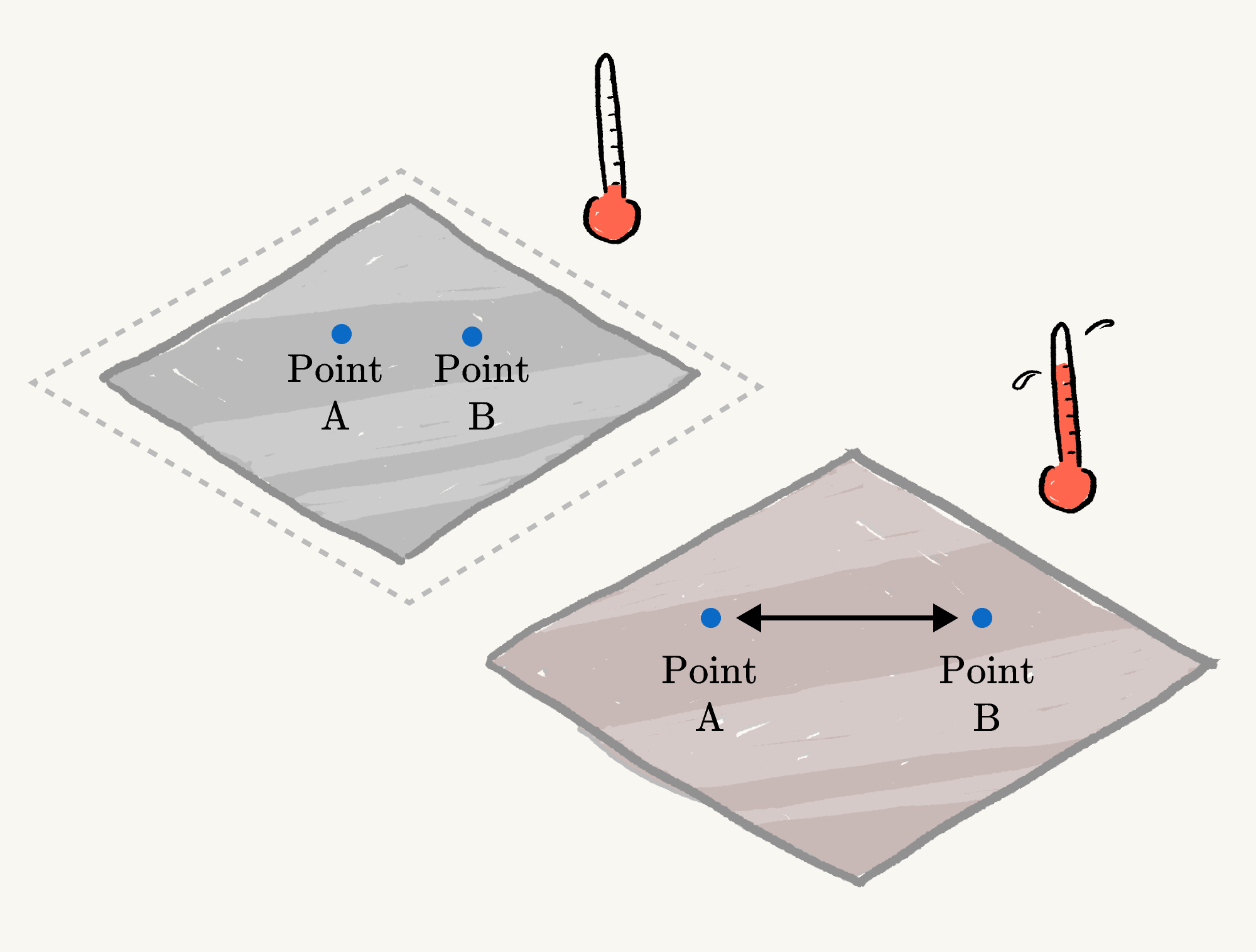## A Different Type of Thermometer

If you want to know the temperature outside, that information is only a click or tap away. Before computers and smartphones, however, you needed to look at an actual thermometer.One style of thermometer is a liquid-in-glass thermometer. These consist of a glass tube filled with a visible liquid (such as mercury or dyed alcohol). The glass tube is marked with different temperatures, and the temperature reading corresponds to the mark closest to the top surface of the liquid.

A thermometer works because matter expands as it gets hotter: at higher temperatures the liquid takes up a larger volume, filling up more of the inside of the glass tube. The marks on the glass tube are calibrated such that the mark for $\SI{0}{\celsius}$ is reached when the liquid is at $\SI{0}{\celsius}$, the mark for $\SI{10}{\celsius}$ is reached when the liquid is at $\SI{10}{\celsius}$, and so on.

Thermal expansion affects all matter, not just liquids. Solids also expand as they get hotter. Unlike a liquid, solids aren't constrained by a container, so they expand along every dimension. When a solid rod is heated, its length increases. Sheets will increase in area, and cubes will increase in volume.In other words, the distance between any two points on the solid increases as its temperature increases.

You might wonder, "If the solid glass tube of the thermometer also gets bigger as it heats up, why does the level of the liquid go up at all?"

It's true that the volume inside the glass tube will increase as it heats up, but the liquid inside expands much faster than the glass (by 10 to 100 times), so it still reaches higher temperature marks as the temperature of the whole thermometer increases.

How much a material expands over a $\SI{1}{\celsius}$ rise in temperature is called its coefficient of thermal expansion. For example, the coefficient of thermal expansion of glass is $\num{5e-6}$ per degree Celsius, while the coefficient of thermal expansion of the liquid inside a thermometer is much higher. This means after a rise of $\SI{1}{\celsius}$, a glass tube's length is multiplied by $1.000005.$

This amount seems tiny, but if glass (or another solid) isn't given room to expand and contract with natural temperature changes in the environment, these small changes can sometimes be enough to cause it to crack or shatter.

# Today's Challenge

While liquid-in-glass thermometers are the most iconic style of thermometer, they're not the only type. Another approach is using a bimetal strip: a strip with two metals that have different coefficients of thermal expansion. The bimetal strip below is made of copper on the left and iron on the right, which have relatively high and low coefficients of thermal expansion, respectively.If we wanted to make a thermometer by putting a pointer at the end of this strip, where should the higher temperature marks go?

×